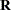REPEATED Statement
REPEATED repeated-effect </ options> ;

The REPEATED statement defines the repeated effect and the residual covariance structure in the mixed model. The residual variance-covariance matrix is denoted as. The repeated-effect is required and consists entirely of classification variables. The levels of the repeated-effect must be different for each observation within a subject in order to avoid the singularmatrix. The SUBJECT= option is required. The data set must be grouped by subject effect.

Table 45.8 Summary of Important REPEATED Statement Options

Option

Description

Construction of Covariance Structure

Defines an effect that specifies heterogeneity in the residual covariance structure

Identifies the subjects in the residual covariance structure

Specifies the residual covariance structure (the default is VC)

Statistical Output

Displays blocks of the estimatedmatrix

Display the Cholesky root (lower) of blocks of the estimatedmatrix

Displays the inverse Cholesky root (lower) of blocks of the estimatedmatrix

Displays the correlation matrix that corresponds to blocks of the estimatedmatrix

Displays the inverse of blocks of the estimatedmatrix

You can specify the following options in the REPEATED statement after a slash (/).

GROUP=effect
GRP=effect

defines an effect that specifies heterogeneity in the residual covariance structure. All observations that have the same level of the GROUP effect have the same covariance parameters. Each new level of the GROUP effect produces a new set of covariance parameters with the same structure as the original group. You should exercise caution in defining the GROUP effect, because strange covariance patterns can result with its misuse. Also, the GROUP effect can greatly increase the number of estimated covariance parameters, which can adversely affect the optimization process.

Continuous variables are permitted as arguments to the GROUP= option. PROC HPMIXED does not sort by the values of the continuous variable; rather, it considers the data to be from a new subject or group whenever the value of the continuous variable changes from the previous observation. Using a continuous variable decreases execution time for models with a large number of subjects or groups and also prevents the production of a large "Class Level Information" table.

R<=value-list>

requests that blocks of the estimatedmatrix be displayed. The first block determined by the SUBJECT= effect is the default displayed block.

The value-list indicates the subjects for which blocks ofare to be displayed. For example, the following statement displays block matrices for the first, third, and fifth persons:

```repeated time / type=un subject=person r=1,3,5;
```

See the PARMS statement for the possible forms of value-list.

RC<=value-list>

displays the Cholesky root of blocks of the estimatedmatrix. The value-list specification is the same as for the R= option.

RCI<=value-list>

displays the inverse Cholesky root of blocks of the estimatedmatrix. The value-list specification is the same as for the R= option.

RCORR<=value-list>

displays the correlation matrix that corresponds to blocks of the estimatedmatrix. The value-list specification is the same as for the R= option.

RI<=value-list>

produces the inverse of blocks of the estimatedmatrix. The value-list specification is the same as for the R= option.

SUBJECT=effect
SUB=effect

identifies the subjects in your mixed model. Complete independence is assumed across subjects; therefore, the SUBJECT= option produces a block-diagonal structure inwith identical blocks. The SUBJECT= option is required. The data set must be grouped by SUBJECT= effect. When the SUBJECT= effect consists entirely of classification variables, the blocks ofcorrespond to observations that share the same level of that effect. These blocks are sorted according to this effect as well.

Continuous variables are permitted as arguments to the SUBJECT= option. PROC HPMIXED does not sort by the values of the continuous variable; rather, it considers the data to be from a new subject or group whenever the value of the continuous variable changes from the previous observation. Using a continuous variable decreases execution time for models with a large number of subjects or groups and also prevents the production of a large "Class Level Information" table.

If you want to model nonzero covariance among all of the observations in your data, specify SUBJECT=INTERCEPT to treat the data as if they are all from one subject. However, be aware that in this case PROC HPMIXED manipulates anmatrix with dimensions equal to the number of observations.

TYPE=covariance-structure

specifies the structure of the residual variance-covariance matrix. The SUBJECT= option defines the blocks of, and the TYPE= option specifies the structure of these blocks. PROC HPMIXED supports the following structures: TYPE=AR(1), TYPE=CHOL, TYPE=UN, and TYPE=VC. The default structure is VC. See the description in the section RANDOM Statement for more information about these covariance structure types.